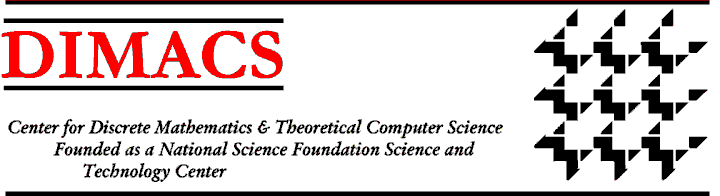# Tung's pageName
Tung Anh, Vu
tung (at) kam.mff.cuni.cz
tv157 (at) dmac.rutgers.edu
Home institution
Faculty of Mathematics and Physics, Charles University
Project
Antipodal monochromatic paths in hypercubes
Research interests
Parameterized and approximation algorithms (preferably both at the same time :) ), computational complexity.

Given an edge 2-coloring of a hypercube Q_n such that each pair of antipodal edges have different color, Norine conjcetures that there always exists a pair of vertices, which can be connected by a monochromatic path. During the programme we will try to answer this conjecture.

## Weekly log

### Week 1

Our group prepared a presentation for the opening week to introduce our topic to the rest of participants.

### Week 2

We tried to extend Dvořák's result. In the paper hypercubes of dimension n are split into subcubes of dimension 3 and he estimates the expected number of switches in each of those cubes of dimension 3. Then he chooses a random antipodal geodesic and using the aforementioned expectations, he glues the cubes of dimension three to obtain his upper bound. We tried to estimate the expected number of switches in cubes of dimension higher than 3, but it turned out to be much more difficult than we expected. In particular there is a lot of dependent events in the cubes. Next week we will try to overcome these dependent events using linearity of expectation.

### Week 3

We try to get a better understanding the average number of switches between all pairs of antipodal vertices in a random (not necessarily antipodal) coloring. We created some programs to help visualise and find “bad colorings”.

### Week 4

We define a switch vector u of a fixed coloring of a Q_n as a vector of length 2^{n-1} where u_i denotes the minimum number of switches between i and its antipodal vertex i'. We define a function f: ℕ → ℝ as the maximum ratio between the sum of the entries of a switch vector and 2^n over all colorings of Q_n.

To list a few values, we know f(1) = 0, f(2) = 1, f(3) = 1. Computationally we know that f(4) = 1.25. We conjecture that f(5) = 1.25 also. We have a proof that function f is non decreasing.

### Week 5

We tried to examine a hypercube of dimension n by splitting it into subcubes of dimension n-1 in n different ways by fixing each coordinate. Unfortunately this did not yield any new results.

We also tried to give a bound on combining two switch vectors of a hypercube of dimension n-1 into a switch vector of a hypercube of dimension n. This also did not yield any new knowledge.

### Week 6

We tried to give a bound on function f for Q_5. We restricted ourselves to the situation when 3 lies in the switch vector of Q_5 and derive consequences of such restriction. We discovered that if the Q_5 contains no subcube Q_3 colored level by level, then we get the desired f(5) ≤ 1.25. However when such Q_3 is present, we cannot bound f(5) yet.

### Week 7 & Week 8

We made our last attempts at computing f(5). Unfortunately none of our attempts yielded anything new.

We started writing our report and preparing final the presentation.

### Week 9

We finished writing our paper and gave a presentation on our group's results.

## Acknowledgements

This work was carried out while the author Tung Anh Vu was a participant in the 2020 DIMACS REU program, supported by CoSP, a project funded by European Union’s Horizon 2020 research and innovation programme, grant agreement No. 823748.